#### You may also like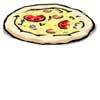### Pizza Portions

My friends and I love pizza. Can you help us share these pizzas equally?### Tweedle Dum and Tweedle Dee

Two brothers were left some money, amounting to an exact number of pounds, to divide between them. DEE undertook the division. "But your heap is larger than mine!" cried DUM...### Sum Equals Product

The sum of the numbers 4 and 1 [1/3] is the same as the product of 4 and 1 [1/3]; that is to say 4 + 1 [1/3] = 4 ï¿½ 1 [1/3]. What other numbers have the sum equal to the product and can this be so for any whole numbers?

# Smaller and Smaller

##### Age 7 to 14Challenge Level

Caroline and Bronya from Tattingstone School sent wonderfully explained solutions to this problem. Caroline drew some diagrams to help her: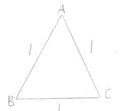Side AB = 1 Perimeter = 3 x 1 = 3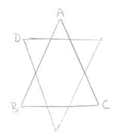Side ADB = 4 x 1/3 = 4/3 Perimeter = 3 x 4/3 = 4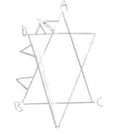Side ADB = 4 x 4/9 = 16/9 Perimeter = 3 x 16/9 = 48/9 = 16/3 = 5 1/3

She explains:

The pattern is every time you add on a new smaller spike you multiply by 4 and divide by 3.

Here, Bronya describes how she approached the problem:

First of all I looked at the perimeter of the equilateral triangle. It was 3 units. Then I looked at the perimeter of the star. If one side is 11/3 units then the perimeter is 4 units. Then the perimeter of the third shape. I looked at a section like this: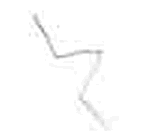Each section = 1/3 + 1/9 unit = 3/9 + 1/9 = 4/9 unit
There are 12 sections so the total perimeter = 4/9 x 12/1 = 48/9
I looked at all the perimeters as ninths:
Perimeter 1 was 27/9
Perimeter 2 was 36/9
Perimeter 3 was 48/9
Each time the perimeter increases by one third.
I think this comes about because in each section a third of the section is added on.

The perimeter of the next shape would be 64/9 because:
48 divided by 3 is 16 therefore increase 48/9 by 16/9
Total would be: 48/9 + 16/9 = 64/9

Thank you to you both - these are very well reasoned solutions. I wonder whether you could generalise to any shape in this series?